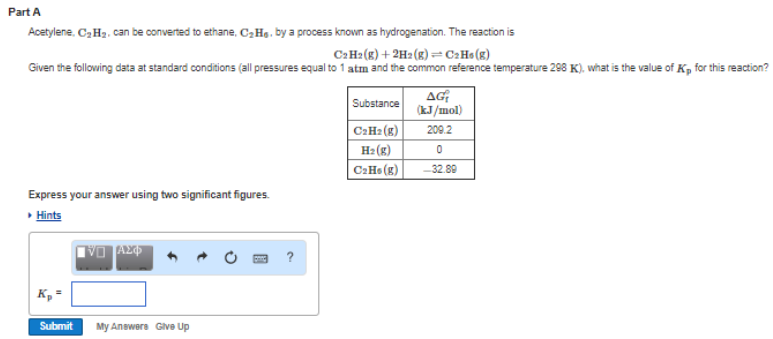# Problem:  Part AAcetylene C2H2 can be convened to ethane. C2H6, by a process known as hydrogenation. The reaction is C2H2 (g) + 2H2 (g) ⇌ C2H5 (g) Given the following data at standard conditions (all pressures equal to 1 atm and the common reference temperature 298 K), what is the value of Kp for this reaction? Express your answer using two significant figures.

###### FREE Expert Solution
79% (102 ratings)###### Problem Details

Part A

Acetylene C2H2 can be convened to ethane. C2H6, by a process known as hydrogenation. The reaction is

C2H2 (g) + 2H2 (g) ⇌ C2H5 (g)

Given the following data at standard conditions (all pressures equal to 1 atm and the common reference temperature 298 K), what is the value of Kp for this reaction?